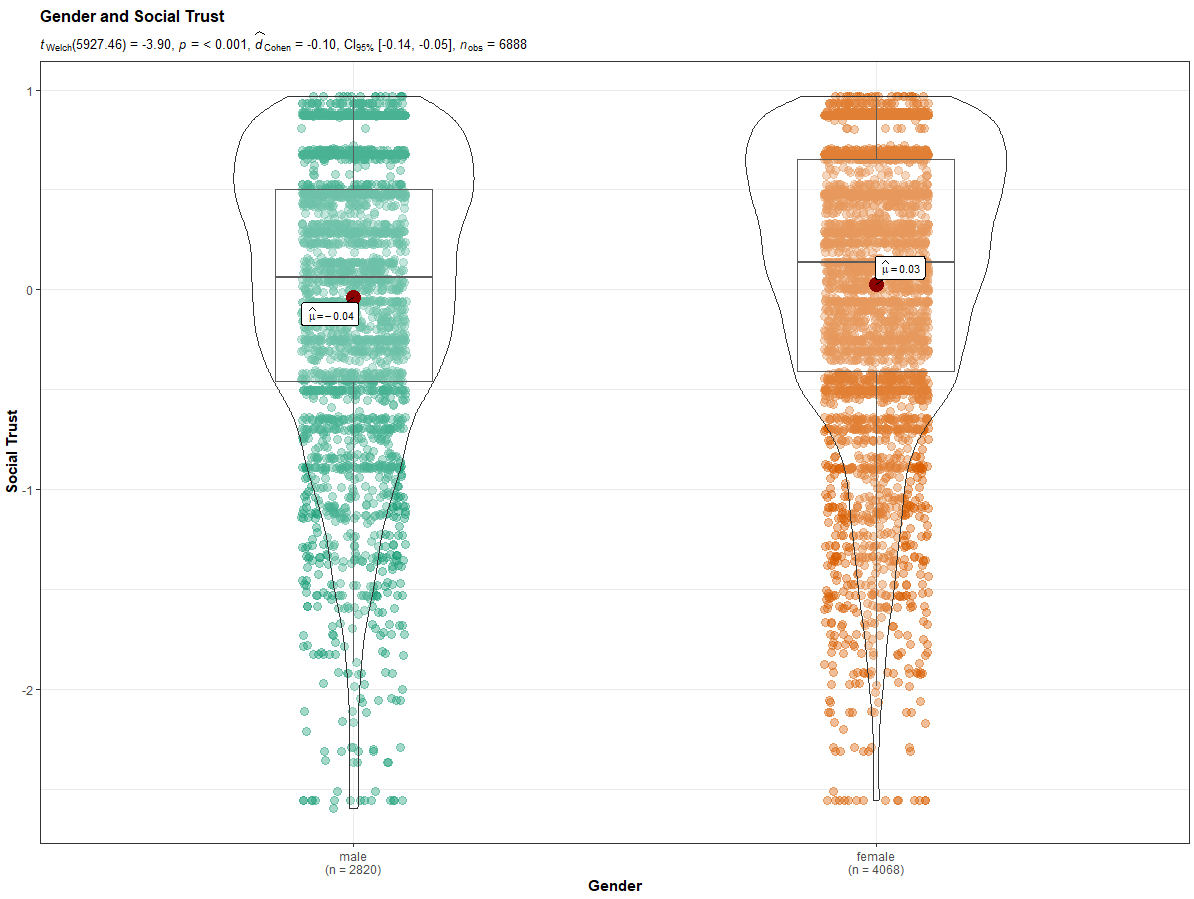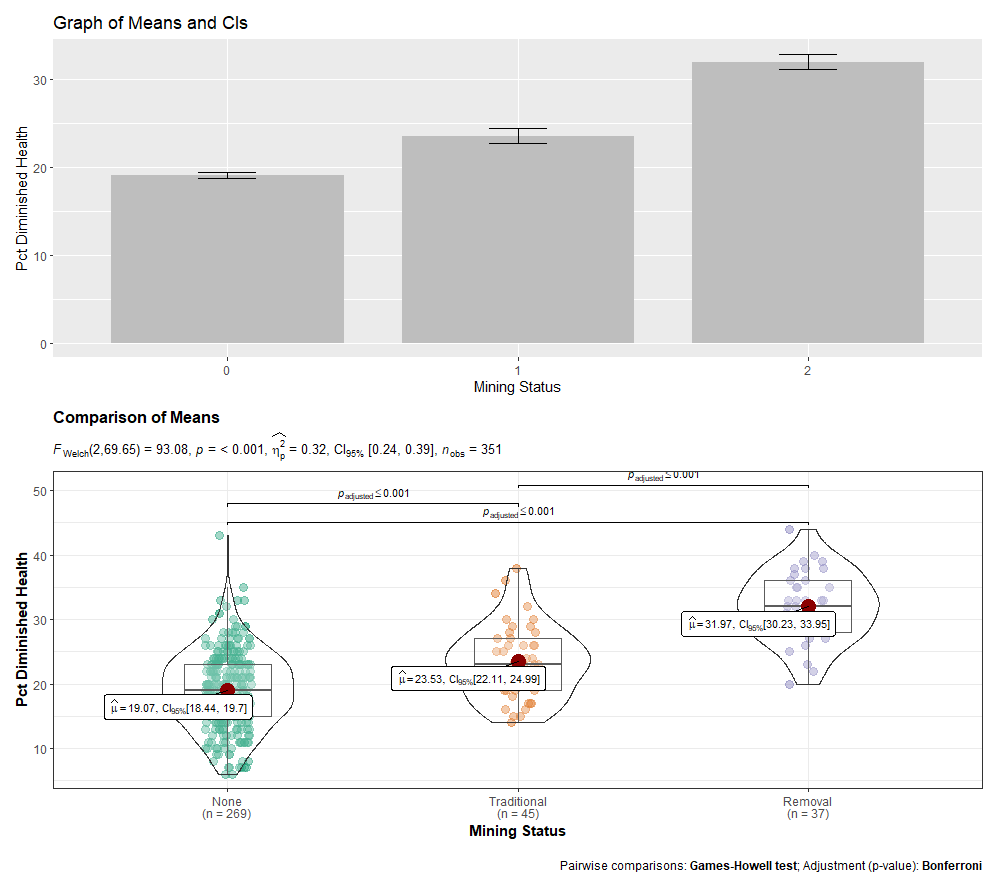# Chapter 6 T-Test (two-sample using groups)

This chapter provides generic code for carrying out a T-Test analysis (two sample using groups). It is recommended that you proceed through the sections in the order they appear. If, however, you want to do a quick analysis, I recommend loading the packages, prepping your data, and then running the ggstatsplot::ggbetweenstats command in section 6.6.

Placeholders that need replacing:

• mydata – name of your dataset
• groupvar – name of your dichotomous grouping variable
• intvar – name of your interval or continuous variable
• label – any titles, axis labels, category labels

## 6.1 Packages Needed for T-Test

This code will check that required packages for this chapter are installed, install them if needed, and load them into your session.

``````req <- substitute(require(x, character.only = TRUE))
libs<-c("effsize", "ggplot2", "ggstatsplot", "patchwork", "moments", "ggpubr")
sapply(libs, function(x) eval(req) || {install.packages(x); eval(req)})``````

## 6.2 Data Prep for T-Test

One of the key preparations you need to make is to declare (classify) your categorical variable as a factor variable. In the generic commands below, the ‘class’ function tells you how R currently sees the variable (e.g., double, factor, character). The second command will reclassify the specified categorical variable as a factor variable.

``````class(mydata\$groupvar) # Will tell you how R currently views the variable (double, factor…)
mydata\$groupvar <- factor(mydata\$groupvar) # Will declare the variable as a factor variable``````

## 6.3 Checking Data for Violations of Assumptions for T-Test

There are generally three assumptions researchers check when conducting ANOVA: 1) Relatively equal group sizes; 2) Relatively equal variances; 3) That the interval or continuous variable is normally distributed. The following generic commands offer insights on each.

### 6.3.1 Group Frequencies for T-Test

``table(mydata\$groupvar)``

### 6.3.2 Checking for Equal Variances for T-Test

Group means and standard deviations (Note: the ‘aggregate’ and ‘by’ functions give you the same results, just in slightly different formats). The var.test function offers a more direct statistical test, performing an F test to compare the variances of the two groups.

``````aggregate(mydata\$intvar, by = list(mydata\$groupvar), FUN = mean, na.rm = TRUE)
aggregate(mydata\$intvar, by = list(mydata\$groupvar), FUN = sd, na.rm = TRUE)

by(mydata\$intvar, mydata\$groupvar, mean, na.rm = TRUE)
by(mydata\$intvar, mydata\$groupvar, sd, na.rm = TRUE)

var.test(intvar ~ groupvar, data = mydata)``````

### 6.3.3 Checking Normality for T-Test

The following generic commands can be used to check for skewness and kurtosis (a skewness of 0 and kurtosis of 3 are considered normal). You can visually inspect the data by looking at a density graph and quantile-quantile (qqplot, which draws a correlation between a sample and a normal distribution; the dots should form a relatively straight 45 degree line if htere is a normal distribution).

``````moments::skewness(mydata\$intvar, na.rm = TRUE)
moments::kurtosis(mydata\$intvar, na.rm = TRUE)
ggpubr::ggdensity(mydata\$intvar, fill = "lightgray")
ggpubr::ggqqplot(mydata\$intvar)``````

Beyond a visual inspection, you can conduct a Shapiro-Wilk’s test of normality, where a p < .05 indicates a non-normal distribution and a p > .05 indicates normally distributed data. This can be a sensitive test, particularly with a large N, so use in conjunction with other information. It is also limited to a sample of 5000.

``shapiro.test(mydata\$intvar)``

## 6.4 T-Test Command (two sample test of group means) and Effect Size

Note that the default is for R to treat the groups as having unequal variances. If this assumption has not been violated, then you may set var.equal = TRUE, as shown below.

``````t.test(intvar ~ groupvar, data = mydata) # The default is for unequal variances
t.test(intvar ~ groupvar, data = mydata, var.equal = TRUE)``````

To calculate Cohen’s d, a measure of effect size, run the following command. In general, the characterizations of effect size are: |0-.2| (small); |.2-.5| (moderate); |>.5| (large)

``effsize::cohen.d(mydata\$intvar, mydata\$groupvar)``

## 6.5 Wilcoxon/Mann-Whitney Rank Sum Test

If you have violated any of the assumptions for t-test, you can run the Wilcoxon/Mann-Whitney Rank Sum Test, a non-parametric test of association.

``wilcox.test(intvar ~ groupvar, data = mydata)``

## 6.6 Graphing Options for T-Test

Boxplots and/or violin plots are probably the most helpful graphs to complement a t-test. The ggplot2 package is the go-to package for most graphing. The ggstatsplot package and ggbetweenstats function, however, provide a one-stop shop for graphing, displaying means, and conducting a t-test. I actually recommend starting with the the ggstatsplot option. You can find helpful webpages on ggstatsplot::ggstatsbetween here, here, and here.

I also encourage you to check out the R Graph Gallery, a website that showcases different graphs and provides their associated code.

Some important arguments that can be change in ggbetweenstats include:

• plot.type = # options include “box,” “violin,” or the default “boxviolin”
• pairwise.comparisons = # Can be set to TRUE or FALSE
• var.equal = # Can be set to TRUE or FALSE (the default)
• mean.ci = # Can be set to TRUE or FALSE (the default)
• effsize.type = # Can be set to “d” for t-tests
``````ggstatsplot::ggbetweenstats(data = mydata, x = groupvar, y = intvar,
effsize.type = "d", mean.ci = TRUE,
pairwise.comparisons = TRUE,
messages = FALSE, bf.message = FALSE,
title = "Title of Graph", xlab = "X-axis label", ylab = "Y-axis label")``````This ggplot command produces a basic boxplot.

``````ggplot2::ggplot(data = mydata, aes(x = groupvar, y = intvar)) +
geom_boxplot() +
labs(title = "Title of Graph", x = "X-axis label", y = "Y-axis label")``````

This version of the ggplot command includes a subcommand for plotting the means on top of the boxplots.

``````ggplot2::ggplot(data = mydata, aes(x = groupvar, y = intvar)) +
geom_boxplot() + stat_summary(fun.y = mean, geom = "point", shape = 8, size = 4, color = "blue", fill = "blue") +
labs(title = "Title of Graph", x = "X-axis label", y = "Y-axis label")``````

This version of the gpplot command includes a subcommand for overlaying a violin plot atop the boxplot.

``````ggplot2::ggplot(data = mydata, aes(x = groupvar, y = intvar)) +
geom_boxplot() + geom_violin() +
labs(title = "Title of Graph", x = "X-axis label", y = "Y-axis label")``````

Lastly, you may consider “patching” your desired graphs together with the patchwork. You’d only need to assign each graph to an object to do so. Here is an example of patching a bar graph and a ggstatsplot graph together (ANOVA example).## 6.7 Consolidated Code for T-Test

Below is the consolidated code from this chapter. One could transfer this code into an empty RScript, which also offers the option of find/replace terms. You can also download this generic t-test RScript file here.

Placeholders that need replacing:

• mydata – name of your dataset
• groupvar – name of your categorical grouping variable
• intvar – name of your interval or continuous variable
• object – whatever you want to call your object(s)), if you generate any
• labels/title – any titles, axis labels, category labels
``````# 5.1 Packages Needed

req <- substitute(require(x, character.only = TRUE))
libs<-c("effsize", "ggplot2", "ggstatsplot", "patchwork", "moments", "ggpubr")
sapply(libs, function(x) eval(req) || {install.packages(x); eval(req)})

# 5.2 Prep Data

class(mydata\$groupvar)
mydata\$groupvar <- factor(mydata\$groupvar)

# 5.3 Checking data for violations of assumptions:

## Group frequencies:

table(mydata\$groupvar)

## Group means and standard deviations. Either the "aggregate" or "by" command works

aggregate(mydata\$intvar, by = list(mydata\$groupvar), FUN = mean, na.rm = TRUE)
aggregate(mydata\$intvar, by = list(mydata\$groupvar), FUN = sd, na.rm = TRUE)
by(mydata\$intvar, mydata\$groupvar, mean, na.rm = TRUE)
by(mydata\$intvar, mydata\$groupvar, sd, na.rm = TRUE)

## Test of equal variances across groups

var.test(intvar ~ groupvar, data = mydata)

## Check for Normality

moments::skewness(mydata\$intvar, na.rm = TRUE)
moments::kurtosis(mydata\$intvar, na.rm = TRUE)
ggpubr::ggdensity(mydata\$intvar, fill = "lightgray")
ggpubr::ggqqplot(mydata\$intvar)

shapiro.test(mydata\$intvar)

# 5.4 T-Test command (two sample test of group means) and Cohen's d

t.test(intvar ~ groupvar, data = mydata) # The default is for unequal variances
t.test(intvar ~ groupvar, data = mydata, var.equal = TRUE)

effsize::cohen.d(mydata\$intvar, mydata\$groupvar)

# 5.5 Wilcoxon/Mann-Whitney Rank Sum Test (non-parametric)

wilcox.test(intvar ~ groupvar, data = mydata)

# 5.6 Graphing Options

## ggstatsplot::ggbetweeenstats is my "go to" option

ggstatsplot::ggbetweenstats(data = mydata, x = groupvar, y = intvar,
effsize.type = "d", mean.ci = TRUE,
pairwise.comparisons = TRUE,
messages = FALSE, bf.message = FALSE,
title = "Title of Graph", xlab = "X-axis label", ylab = "Y-axis label")

## ggplot commands

ggplot2::ggplot(data = mydata, aes(x = groupvar, y = intvar)) +
geom_boxplot() +
labs(title = "Title of Graph", x = "X-axis label", y = "Y-axis label")

ggplot2::ggplot(data = mydata, aes(x = groupvar, y = intvar)) +
geom_boxplot() + stat_summary(fun.y = mean, geom = "point",
shape = 8, size = 4, color = "blue", fill = "blue") +
labs(title = "Title of Graph", x = "X-axis label", y = "Y-axis label")

ggplot2::ggplot(data = mydata, aes(x = groupvar, y = intvar)) +
geom_boxplot() + geom_violin() +
labs(title = "Title of Graph", x = "X-axis label", y = "Y-axis label")
``````